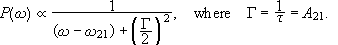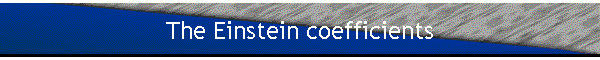The transition probability Pif(t,w) for an atom placed into an electromagnetic plane wave with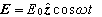and angular frequency w near wfi is in the electric dipole approximation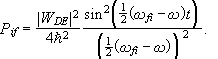Here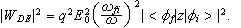The incident radiation, however is often not monochromatic.  Let I(w)dw be the intensity of the radiation in the interval between w and w+dw.  Assume the incident radiation is incoherent. Then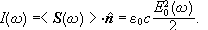The total transition probability can now be found by integrating the transition probability induced by the radiation in each frequency interval.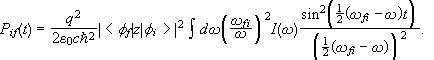If I(w) varies little in the interval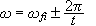and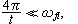then we may evaluate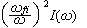at w=wfi and treat it as a constant.  We then obtain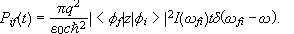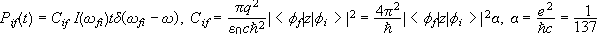The transition probability per unit time is given by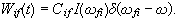The transition probability per unit time is proportional to the intensity of the radiation for induced transitions.

We can calculate induced transition probabilities using time-dependent perturbation theory, but as long as we treat the electromagnetic field classically, we cannot calculate the probability for spontaneous emission of a photon this way.

We can however avoid this problem by making statistical arguments.  Consider two atomic states of energy E1 and E2.  Let E2>E1.  Assume the atom is bathed in radiation of energy density u(w).  Transitions between these states can take place in three different ways.

• (1)  spontaneous, 2 to 1, with probability A21 per unit time;
• (2)  induced, 2 to 1, with probability B21u(w12) per unit time;
• (3)  induced, 1 to 2, with probability B12u(w12) per unit time.

A21, B21, and B12 are called the Einstein coefficients.

In a cavity in thermal equilibrium the probabilities that states 1 and 2 are occupied are exp(-E1/(KT)) and exp(-E2/(kT)) respectively, and in equilibrium the probability of up transitions must exactly balance the probability of down transitions. We therefore need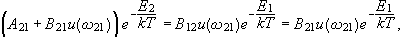since B12=B21 is given by C12 from above, averaged over all polarizations and all directions of incidence of the plane wave.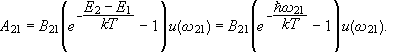Since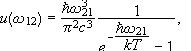(Planck’s law),

we have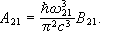#### Problem:

(a) Consider first a two level system in thermal equilibrium with radiation of energy densityUse this information to derive the relations among Einstein's A and B coefficients which measure the spontaneous and the induced transition probabilities, respectively, between energy levels Ea and Eb.

(b) Now consider an atom in an electromagnetic field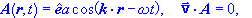with Hamiltonian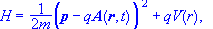where -q is the charge of the electron.  The energy density in the field is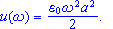Neglecting terms in A2, calculate the probability for absorption of radiation in the dipole approximation.  Express your answer in terms of matrix elements of the form <b|r|a>.

(c) Relate the lifetime of an excited state to the A coefficient and calculate the shape of the intensity distribution ( as a function of w ) observed as a consequence of spontaneous emission.

• Solution:

(a)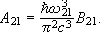(This was shown above.)

(b)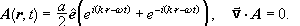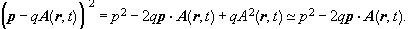The component of p in the direction of A commutes with AA is perpendicular to the direction of propagation but depends on the coordinate in the direction of propagation.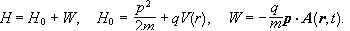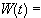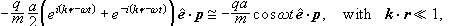( dipole approximation ).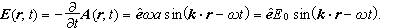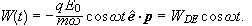Therefore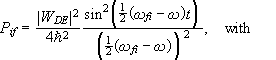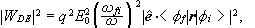with i = b and f = a.  (See previous module.)

This is what is required for part (b) of the problem.  Let us expand and assume that the incident radiation is not monochromatic.  Let I(w)dw be the intensity of the radiation in the interval between w and dw.  Assume the incident radiation is incoherent and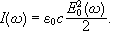Then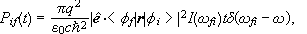and the transition probability per unit time is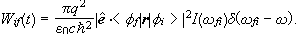Let us expand even further and assume that the radiation is isotropic.  In order to find the total rate induced by an isotropic field, it is necessary to sum this expression over the two possible states of polarization for each direction of propagation and to integrate over all directions of propagation.  The two polarization vectors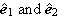and the unit vector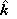form a triplet of orthogonal unit vectors.  For any fixed vector r we have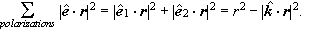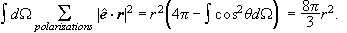Now let us relate I(wfi) to u(wfi) for the isotropic field.  For a particular polarization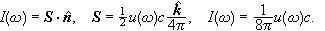The factor of ½ is needed because u(w) contains two linearly independent polarizations.  We now have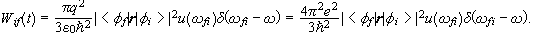(c)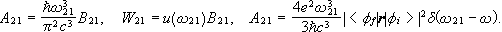A21 is the spontaneous transition rate.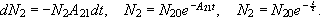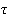is the lifetime,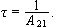Since the energies of excited states are not infinitely sharp, the emission lines have Lorentzian shapes. We write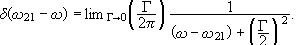Therefore